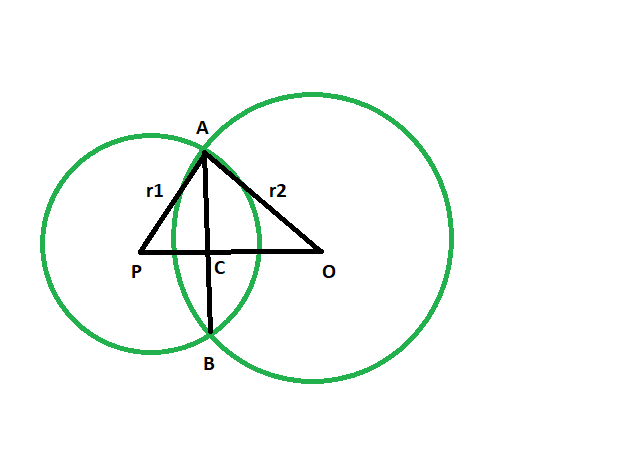# Distance between centers of two intersecting circles if the radii and common chord length is given

Given are two circles, with given radii, which intersect each other and have a common chord. The length of the common chord is given. The task is to find the distance between the centres of the two circles.

Examples:

```Input:  r1 = 24, r2 = 37, x = 40
Output: 44

Input: r1 = 14, r2 = 7, x = 10
Output: 17
```Approach:

• let the length of common chord AB = x
• Let the radius of the circle with centre O is OA = r2
• Radius of circle with centre P is AP = r1
• From the figure, OP is perpendicular AB
AC = CB
AC = x/2 (Since AB = x)
• In triangle ACP,
AP^2 = PC^2+ AC^2 [By Pythagoras theorem]
r1^2 = PC^2 + (x/2)^2
PC^2 = r1^2 – x^2/4
• Consider triangle ACO
r2^2 = OC^2+ AC^2[By Pythagoras theorem]
r2^2 = OC^2+ (x/2)^2
OC^2 = r2^2 – x^2/4
• From the figure, OP = OC + PC
OP = √( r1^2 – x^2/4 ) + √(r2^2 – x^2/4)
• Distance between the centres = sqrt((radius of one circle)^2 – (half of the length of the common chord )^2) + sqrt((radius of the second circle)^2 – (half of the length of the common chord )^2)

Below is the implementation of the above approach:

## C++

 `// C++ program to find ` `// the distance between centers ` `// of two intersecting circles ` `// if the radii and common chord length is given ` ` `  `#include ` `using` `namespace` `std; ` ` `  `void` `distcenter(``int` `r1, ``int` `r2, ``int` `x) ` `{ ` `    ``int` `z = ``sqrt``((r1 * r1) ` `                 ``- (x / 2 * x / 2)) ` `            ``+ ``sqrt``((r2 * r2) ` `                   ``- (x / 2 * x / 2)); ` ` `  `    ``cout << ``"distance between the"` `         ``<< ``" centers is "` `         ``<< z << endl; ` `} ` ` `  `// Driver code ` `int` `main() ` `{ ` `    ``int` `r1 = 24, r2 = 37, x = 40; ` `    ``distcenter(r1, r2, x); ` `    ``return` `0; ` `} `

## Java

 `// Java program to find ` `// the distance between centers ` `// of two intersecting circles ` `// if the radii and common chord length is given ` `import` `java.lang.Math;  ` `import` `java.io.*; ` ` `  `class` `GFG { ` `     `  `static` `double` `distcenter(``int` `r1, ``int` `r2, ``int` `x) ` `{ ` `    ``double` `z = (Math.sqrt((r1 * r1) ` `                ``- (x / ``2` `* x / ``2``))) ` `            ``+ (Math.sqrt((r2 * r2) ` `                ``- (x / ``2` `* x / ``2``))); ` ` `  `    ``System.out.println (``"distance between the"` `+ ` `                        ``" centers is "``+ (``int``)z ); ` `    ``return` `0``; ` `} ` ` `  `// Driver code ` `public` `static` `void` `main (String[] args)  ` `{ ` `    ``int` `r1 = ``24``, r2 = ``37``, x = ``40``; ` `    ``distcenter(r1, r2, x); ` `} ` `} ` ` `  `// This code is contributed by jit_t. `

## Python3

 `     `  `# Python program to find ` `# the distance between centers ` `# of two intersecting circles ` `# if the radii and common chord length is given ` ` `  `def` `distcenter(r1, r2, x): ` `    ``z ``=` `(((r1 ``*` `r1) ``-` `(x ``/` `2` `*` `x ``/` `2``))``*``*``(``1``/``2``)) ``+``\ ` `    ``(((r2 ``*` `r2)``-` `(x ``/` `2` `*` `x ``/` `2``))``*``*``(``1``/``2``)); ` ` `  `    ``print``(``"distance between thecenters is "``,end``=``""); ` `    ``print``(``int``(z)); ` ` `  `# Driver code ` `r1 ``=` `24``; r2 ``=` `37``; x ``=` `40``; ` `distcenter(r1, r2, x); ` ` `  `# This code has been contributed by 29AjayKumar `

## C#

 `// C# program to find ` `// the distance between centers ` `// of two intersecting circles ` `// if the radii and common chord length is given ` `using` `System; ` ` `  `class` `GFG ` `{ ` `         `  `static` `double` `distcenter(``int` `r1, ``int` `r2, ``int` `x) ` `{ ` `    ``double` `z = (Math.Sqrt((r1 * r1) ` `                ``- (x / 2 * x / 2))) ` `            ``+ (Math.Sqrt((r2 * r2) ` `                ``- (x / 2 * x / 2))); ` ` `  `    ``Console.WriteLine(``"distance between the"` `+ ` `                        ``" centers is "``+ (``int``)z ); ` `    ``return` `0; ` `} ` ` `  `// Driver code ` `static` `public` `void` `Main () ` `{ ` `    ``int` `r1 = 24, r2 = 37, x = 40; ` `    ``distcenter(r1, r2, x); ` `} ` `} ` ` `  `// This code is contributed by jit_t `

Output:

```distance between the centers is 44
```

My Personal Notes arrow_drop_upCheck out this Author's contributed articles.

If you like GeeksforGeeks and would like to contribute, you can also write an article using contribute.geeksforgeeks.org or mail your article to contribute@geeksforgeeks.org. See your article appearing on the GeeksforGeeks main page and help other Geeks.

Please Improve this article if you find anything incorrect by clicking on the "Improve Article" button below.

Improved By : jit_t, 29AjayKumar

Article Tags :
Practice Tags :

Be the First to upvote.

Please write to us at contribute@geeksforgeeks.org to report any issue with the above content.